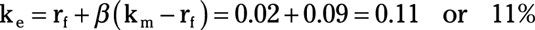##### Behavioral Economics For Dummies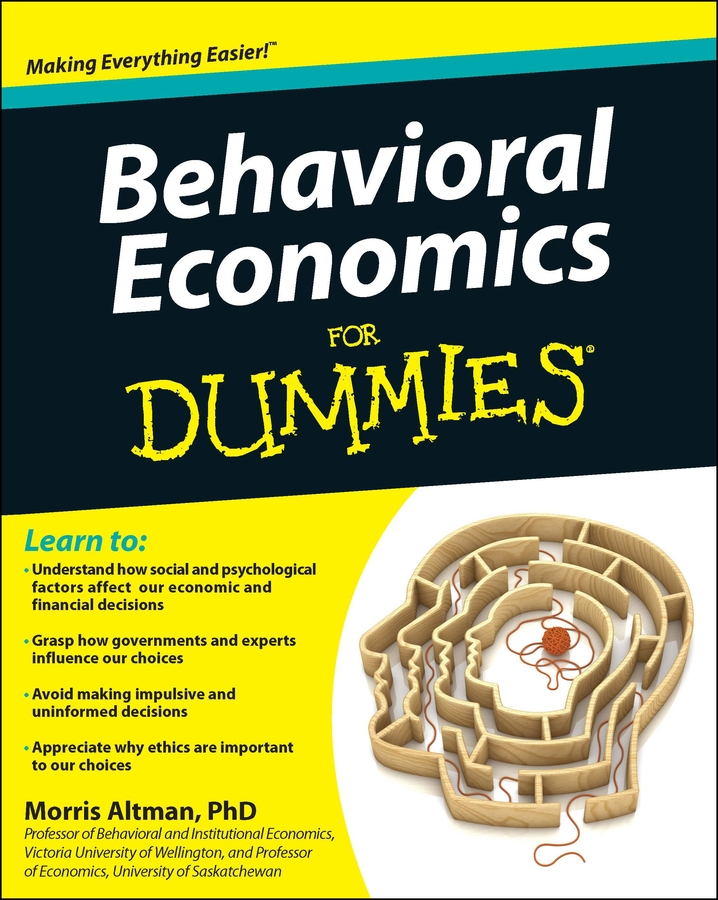The cost of capital of using internal funds is not as straightforward as it would be when borrowing money. Internal funds represent using equity — either the firm’s or the firm’s owner’s financial resources — to finance the project. However, internal funds also cost you — even if you contribute those funds.

The opportunity cost of funds you invest in the firm is the interest you could have earned if you had invested those funds elsewhere. This cost is very real, and your investment project has to generate enough cash to offset this lost opportunity.

Because the cost of using internally generated funds or equity is the lost opportunity for you to invest these funds in the next best alternative, you must use a method that estimates the return the next best alternative generates. Typically, one of three methods — risk-premium, dividend-valuation, or capital-asset pricing — is used to determine the cost of internal or equity capital.

The risk premium method assumes that you incur some additional risk in the investment. This method’s cost estimation uses a risk-free rate of return, rf, plus an additional risk premium, rp, or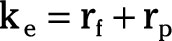where ke is the cost of equity capital. It’s common to use the U.S. Treasury Bill rate as the risk-free rate of return (rf).

The risk premium (rp) that’s added to the risk-free rate of return has two components. First, it includes the difference between the interest rate on company bonds as compared to the U.S. Treasury Bill rate. Second, it includes an additional premium reflecting the added risk of owning the specific company’s stock instead of its bonds.

The risk premium has historically averaged four percent; however, this amount varies substantially among companies and over different length time periods.

## Dividend-valuation method

The dividend valuation method uses shareholder attitudes to determine the cost of equity capital. Shareholder wealth is a function of dividends and changing stock prices. Therefore, a shareholder’s rate of return is a function of the ratio of the dividend, D, divided by the stock price per share, P, plus the expected or historic earnings growth, g. Using this shareholder return as the cost of equity capital results in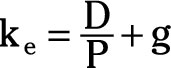Your firm’s stock currently sells at \$24.00 a share and the current annual dividend is \$0.96. Your firm’s historic growth rate is 5 percent. Given this information, use the following steps to calculate the cost of equity capital by using the dividend-valuation method:

1. Determine the ratio of D/P.

This ratio determines the rate of return your invested funds earn through dividends.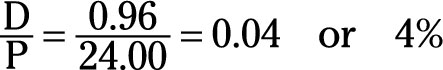2. Add the historic growth rate to the D/P ratio.

This determines the cost of equity capital by including the anticipated growth in the stock’s price.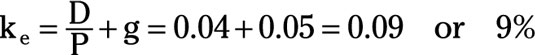## Capital-asset-pricing method

The third method for estimating the cost of equity capital is the capital-asset-pricing method. This method incorporates a risk premium for variability in a company’s return. The risk premium is higher for stocks whose returns are more variable, and lower for stocks with stable returns. The formula used to determine the cost of equity capital using the capital-asset-pricing method is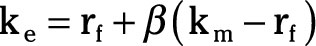where rf is the risk-free return, km is an average stock’s return, and â measures the variability in the specific firm’s common stock return relative to the variability in the average stock’s return.

If â equals 1, the firm has average variability or risk. â values greater than 1 indicate higher than average variability or risk while values less than 1 indicate below average risk. The term â(kmrf) gives the risk premium for holding the firm’s common stock.

The return on U.S. Treasury bills is 2 percent and the average return on stock is 7 percent. Variability in your firm’s stock return relative to variability in the average stock’s return is 1.8. Given this information, the cost of equity capital using the capital-asset-pricing method is calculated through the following steps:

1. Determine the risk premium.

The risk premium is determined by subtracting the risk-free rate of return from the average stock’s return and multiplying by â.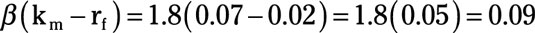2. Add the risk premium to the risk-free rate of return.

This addition determines the cost of equity capital by including the risk premium.